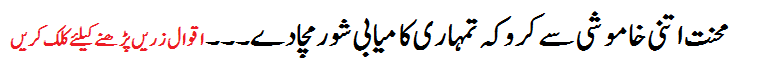# F.Sc Part-1 Math (Chapter 06: Sequences and Series)Chapter No.6 (Sequences And Series)
exercises Of this chapter have following topics:
• Exercise No 6.1 (Types of Sequences)
• Exercise No 6.2 (Arithmetic Progression A.P.)
• Exercise No 6.3 (Aritmetic Mean A.M.)
• Exercise No 6.4 (Series)
• Exercise No 6.5 (World Problems on A.P.)
• Exercise No 6.6 (Geometric Progression G.P.)
• Exercise No 6.7 (Geometric Means G.M)
• Exercise No 6.8 (The Infinite Geometric Series)
• Exercise No 6.9 (World Problems on G.P.)
• Exercise No 6.10 (Solutions of Partial Fractions)
• Exercise No 6.11 (Non-Repeated irreducible quadratic factors)